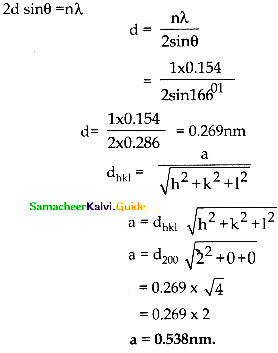Tamilnadu State Board New Syllabus Samacheer Kalvi 12th Chemistry Guide Pdf Chapter 6 Solid State Text Book Back Questions and Answers, Notes.

## Tamilnadu Samacheer Kalvi 12th Chemistry Solutions Chapter 6 Solid State

### 12th Chemistry Guide Solid State Text Book Questions and Answers

Part – I Text Book Evaluation

Question 1.
Graphite and diamond are ………..
(a) Covalent and molecular crystals
(b) ionic and covalent
(c) both covalent crystals
(d) both molecular crystals
(c) both covalent crystalsQuestion 2.
An ionic compound Ax By crystallizes in fee type crystal structure with B ions at the centre of each face and A ion occupying centre of the cube, the correct formula of A B is ………..
(a) AB
(b) AB3
(c) A3B
(d) A8B6
(b) AB3

Question 3.
The rano of close packed atoms to tetrahedral hole in cubic packing is ………..
(a) 1:1
(b) 1:2
(c) 2:1
(d) 1:4
(b) 1:2

Question 4.
Solid CO2 is an example of ………..
(a) Covalent solid
(b) metallic solid
(c) molecular solid
(d) ionic solid
(c) molecular solidQuestion 5.
Assertion: monoclinic sulphur is an example of monoclinic crystal system.
Reason: for a monoclinic system, a $$\neq$$ b $$\neq$$ c and α = γ = 90° , β $$\neq$$ 90°.
(a) Both assertion and reason are true and reason is the correct explanation of assertion.
(b) Both assertion and reason are true but reason is not the correct explanation of assertion.
(c) Assertion is true but reason is false.
(d) Both assertion and reason are false.
(a) Both assertion and reason are true and reason is the correct explanation of assertion

Question 6.
In calcium fluoride, having the flurite structure the coordination number of Ca2+ ion and F Ion are ………..
(a) 4 and 2
(b) 6 and 6
(c) 8 and 4
(d) 4 and 8
(c) 8 and 4

Question 7.
The number of unit cells in 8gm of an element X (atomic mass 40) which crystallizes in bcc pattern is (NA is the Avogadro number)
(a) 6.023 × 1023
(b) 6.023 × 1022
(c) 60.23 × 1023
(d) $$\left( \frac { 6.023\times { 10 }^{ 23 } }{ 8\times 40 } \right)$$
(b) 6.023 × 1022

Question 8.
In a solid atom M occupies ccp lattice and $$\left( \frac { 1 }{ 3 } \right)$$ of tetrahedral voids are occupied by atom N. Find the formula of solid formed by M and N.
(a) MN
(b) M3N
(C) MN3
(d) M3N2
(d) M3N2Question 9
The ionic radii of A+ and B- are 0.98 × 10-10 m and 1.81 × 10-10 m , the coordination number of each ion in AB is ………..
(a) 8
(b) 2
(c) 6
(d) 4
(c) 6

Question 10.
CsCl has bcc arrangement, its unit cell edge length is 400pm, its inter atomic distance is ………..
(a) 400pm
(b) 800pm
(c) $$g\sqrt { 3 }$$ × 100pm
(d) $$\left( \frac { \sqrt { 3 } }{ 2 } \right)$$ × 400 pm
(d) $$\left( \frac { \sqrt { 3 } }{ 2 } \right)$$ × 400 pm

Quesiton 11.
A solid compound XY has NaCl structure, if the radius of the cation is 100pm , the radius of the anion will be ………..
(a) $$\left( \frac { 100 }{ 0.414 } \right)$$
(b) $$\left( \frac { 0.732 }{ 100 } \right)$$
(c) 100 × 0.414
(d) $$\left( \frac { 0.414 }{ 100 } \right)$$
(a) $$\left( \frac { 100 }{ 0.414 } \right)$$Question 12.
The vacant space in bcc lattice unit cell is ………..
(a) 48%
(b) 23%
(c) 32%
(d) 26%
(c) 32%

Question 13.
The radius of an atom is 300pm, if it crystallizes in a face centered cubic lattice, the length of the edge of the unit cell is ………..
(a) 488.5pm
(b) 848.5pm
(c) 884.5pm
(d) 484.5pm
(b) 848.5pm

Question 14.
The fraction of total volume occupied by the atoms in a simple cubic is ………..
(a) $$\left( \frac { \pi }{ 4\sqrt { 2 } } \right)$$
(b) $$\left( \frac { \pi }{ 6 } \right)$$
(c) $$\left( \frac { \pi }{ 4 } \right)$$
(d) $$\left( \frac { \pi }{ 3\sqrt { 2 } } \right)$$
(b) $$\left( \frac { \pi }{ 6 } \right)$$

Question 15.
The yellow colour in NaCl crystal is due to ………..
(a) excitation of electrons in F centers
(b) reflection of light from Cl- ion on the surface
(c) refraction of light from Na+ ion
(d) all of the above
(a) excitation of electrons in F centersQuestion 16.
If ’a’ stands for the edge length of the cubic system; sc ,bcc, and fcc. Then the ratio of radii of spheres in these systems wilL be respectively.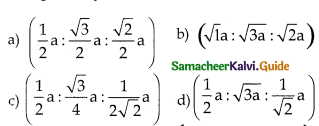$$\left(\frac{1}{2} a: \frac{\sqrt{3}}{4} a: \frac{1}{2 \sqrt{2}} a\right)$$

Question 17.
If a is the length of the side of the cube, the distance between the body centered atom and one comer atom in the cube will be ………..
(a) $$\left( \frac { 2 }{ \sqrt { 3 } } \right) a$$
(b) $$\left( \frac { 4 }{ \sqrt { 3 } } \right) a$$
(c) $$\left( \frac { \sqrt { 3 } }{ 4 } \right) a$$
(d) $$\left( \frac { \sqrt { 3 } }{ 2 } \right) a$$
(d) $$\left( \frac { \sqrt { 3 } }{ 2 } \right) a$$

Question 18.
Potassium has a bcc structure with nearest neighbor distance 4.52 A. its atomic weight is 39. Its density will be ………..
(a) 915 kg m-3
(b) 2142 kg m-3
(c) 452 kg m-3
(d) 390 kg m-3
(a) 915 kg m-3

Question 19.
Schottky defect in a crystal is observed when ………..
(a) unequal number of anions and anions are missing from the lattice
(b) equal number of anions and anions are missing from the lattice
(e) an ion leaves its normal site and occupies an interstitial site
(d) no ion is missing from its lattice.
(b) equal number of anions and anions are missing from the latticeQuestion 20.
The cation leaves its normal position in the crystal and moves to some interstitial position, the defect in the crystal is known as ………..
(a) Schottky defect
(b) F center
(c) Frenkel defect
(d) non-stoichiometric defect
(c) Frenkel defect

Question 21.
Assertion – due to Frenkel defect, density of the crystalline solid decreases.
Reason – in Frenkel defect cation and anion leaves the crystal.
(a) Both assertion and reason are true and reason is the correct explanation of assertion.
(b) Both assertion and reason are true but reason is not the correct explanation of assertion.
(c) Assertion is true but reason is false.
(d) Both assertion and reason are false
(d) Both assertion and reason are false

Question 22.
The crystal with a metal deficiency defect is ………..
(a) NaCI
(b) FeO
(c) ZnO
(a) KCI
(b) FeO

Question 23.
A two dimensional solid pattern formed by two different atoms X and Y is shown below. The black and white squares represent atoms X and Y respectively. The simplest formula for the compound based on the unit cell from the pattern is ………..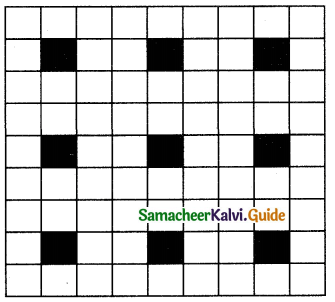(a) XY8
(b) X4Y9
(c) XY2
(d) XY4
(a) XY8

Question 1.
Define unit cell.
A basic repeating structural unit of a crystalline solid is called a unit ccli.Question 2.
Give any three characteristics of ionic crystals.

1. Ionic solids have high melting points.
2. These solids do not conduct electricity, because the ions are fixed in their lattice positions.
3. They do conduct electricity in a molten state (or) when dissolved in water because the ions are free to move in the molten state or solution.

Question 3.
Differentiate crystalline solids and amorphous solids.
Crystalline solids:

1. A long-range orderly arrangement of constituents
2. Definite shape
3. Generally, crystalline solids are anisotropic in nature
4. They are true solids
5. Definite Heat of fusion
6. They have sharp melting points.
7. Examples: NaCl, diamond etc.,

Amorphous solids:

1. The short-range, random arrangement of constituents
2. Irregular shape
3. They are isotropic like liquids
4. They are considered as pseudo solids (or) supercooled liquids
5. Heat of fusion is not definite
6. Gradually soften over a range of temperatures and so can be moulded.
7. Examples: Rubber, plastics, glass etc

Question 4.
Classify the following solids.
a) P4
b) Brass
c) Diamond
d) NaCI
e) iodine
P4 – Molecular solid
Brass – Metallic solid
Diamond –
NaCl – Ionic solid
Iodine – Molecular solidQuestion 5.
Explain briefly seven types of unit cell.
Seven types of unit cell:Cubic – NaCl
Tetragonal – TiO2
Orthorhombic – BaSO4
Hexagonal – ZnO
Monoclinic – PbCrO4
Triclinic – H3BO3
Rhombohedral – Cinnabar Cubic

They differ in the arrangements of their crystallographic axes and angles. Corresponding to the above seven, Bravis defined 14 possible crystal system as shown in the figure.

Question 6.
Distinguish between hexagonal close packing and cubic close packing.
Hexagonal close-packing

1. aba arrangement
2. In this case, the spheres of the third layer are exactly aligned with those of the first layer
3. In HCP, tetrahedral voids of the second layer may be covered by the spheres of the third layer

Cubic close packing:

1. abc arrangement
2. In this case, the spheres of the third layer are not aligned with those of the first layer or second layer. Only when the fourth layer is placed, its spheres are aligned with the first layer.
3. In cep third layer may be placed above the second layer in a manner such that its sphere covers the octahedral voidsQuestion 7.
Distinguish tetrahedral and octahedral voids.
Tetrahedral void

1. A single triangular void in a crystal is surrounded by four (4) spheres and is called a tetrahedral void
2. A sphere of second layer is above the void of the first layer, a tetrahedral void is formed
3. This constitutes four spheres, three in the lower and one in upper layer. When the centres of these four spheres are joined a tetrahedron is formed
4. The radius of the sphere which can be accommodated in an octahedral hole without disturbing the structure should not exceed 0.414 times that of the structure forming sphere
5. Radius of an tetrahedral void $$\frac { r }{ R }$$ = 0.225

Octahedral void

1. A double triangular void like c is surrounded by six(6) spheres and is called an octahedral void
2. The voids in the first layer are partially covered by the spheres of layer now such a void is called a octahedral void
3. This constitutes six spheres, three in the lower layer and three in the upper layer. When the centers of these six spheres are joined an octahedron is formed
4. The sphere which can be placed in a tetrahedral hole without disturbing the close-packed structure should not have a radius larger than 0.225 times the radius of the sphere-forming the structure
5. Radius of a octahedral void $$\frac { r }{ R }$$ = 0.414

Question 8.
What are point defects?
If the deviation occurs due to missing atoms, displaced atoms or extra atoms the imperfection is named as a point defect. Such defects arise due to imperfect packing during the original crystallisation or they may arise from thermal vibrations of atoms at elevated temperatures.Question 9.
Explain Schottky defect.
Schottky defect arises due to the missing of equal number of cations and anions from the crystal lattice. This effect does not change the stoichiometry of the crystal.Ionic solids in which the cation and anion are of almost of similar size show schottky defect.
Example: NaCl.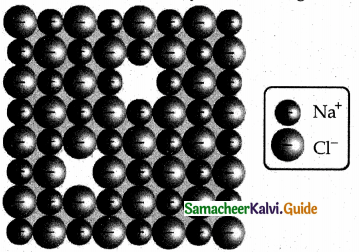Presence of large number of schottky defects in a crystal, lowers its density. For example, the theoretical density of vanadium monoxide (VO) calculated using the edge length of the unit cell is 6.5 g cm-3 but the actual experimental density is 5.6 gcm3. It indicates that there is approximately 14% Schottky defect in VO crystal. Presence of Schottky defect in the crystal provides a simple way by which atoms or ions can move within the crystal lattice.

Question 10.
Write short note on metal excess and metal deficiency defect with an example. Metal excess defect.
Metal excess defect arises due to the presence of more number of metal ions as compared to anions.Alkali metal halides NaCl, KCl show this type of defect.The electrical neutrality of the crystal can be maintained by the presence of anionic vacancies equal to the excess metal ions (or) by the presence of extra cation and electron present in interstitial position.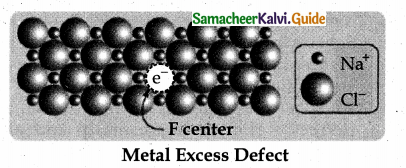For example, when NaCl crystals are heated in the presence of sodium vapour, Na+ ions are formed and are deposited on the surface of the crystal. Chloride ions (Cl) diffuse to the surface from the lattice point and combines with Na+ ion.

The electron lost by the sodium vapour diffuse into the crystal lattice and occupies the vacancy created by the Clions. Such anionic vacancies which are occupied by unpaired electrons are called F centers. Hence, the formula of NaCl which contains excess Na+ ions can be written as Na1+xCl.

Metal deficiency defect:
Metal deficiency defect arises due to the presence of less number of cations than the anions. This defect is observed in a crystal in which, the cations have variable oxidation states. For example, in FeO crystal, some of the Fe2+ ions are missing from the crystal lattice.

To maintain the electrical neutrality, twice the number of other Fe2+ ions in the crystal is oxidized to Fe3+ ions. In such cases, overall number of Fe22+ and Fe3+ ions is less than the O2- ions. It was experimentally found that the general formula of ferrous oxide is FexO, where x ranges from 0.93 to 0.98.Question 11.
Calculate the number of atoms in a fee unit cell.
Number of atoms in a fee unit cell,
= $$\frac { { N }_{ c } }{ 8 }$$ + $$\frac { { N }_{ f } }{ 2 }$$
= $$\frac { 8 }{ 8 }$$ + $$\frac { 6 }{ 2 }$$ = 1 + 3 = 4Question 12.
Explain AAAA and ABABA and ABCABC type of three dimensional packing with the help of neat diagram.
1. AAAA type of three dimensional packing
This type of three dimensional packing arrangements can be obtained by repeating the AAAA type two dimensional arrangements in three dimensions, i.e., spheres in one layer sitting directly on the top of those in the previous layer so that all layers are identical.

All spheres of different layers of crystal are perfectly aligned horizontally and also vertically, so that any unit cell of such arrangement as simple cubic structure as shown in fig.In simple cubic packing, each sphere is in contact with 6 neighbouring spheres – Four in its own layer, one above and one below and hence the coordination number of the sphere in simple cubic arrangement is 6.

2. ABABA type of three dimensional packing:
In this arrangement, the spheres in the first layer (A type) are slightly separated and the second layer is formed by arranging the spheres in the depressions between the spheres in layer A as shown in figure.

The third layer is a repeat of the first. This pattern ABABAB is repeated throughout the crystal. In this arrangement, each sphere has a coordination number of 8, four neighbors in the layer above and four in the layer below.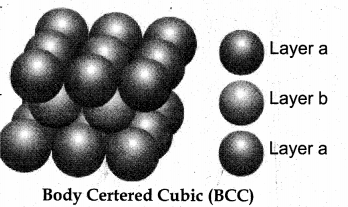3. ABCABC type of three dimensional packing:
In this arrangement (FCC) second layer spheres are arranged at the dips of first layer. Third layer spheres are arranged in a manner such that it cover the octahedral void. Then no longer third layer is similar to first or second layer.

Third layer gives different arrangement. Fourth layer spheres are similar to first layer. If the first, second and third layer are represented as A,B,C then this type of packing gives the arrangement of layers as ABCABC… (i.e.,), the first three layers do not resemble first, second and third layers respectively and the sequence is repeated.

with the addition of more layers. In this arrangement atoms occupy 74% of the available space and thus has 26% vacant space. The coordination number is 12. Voids – The empty spaces between the three dimensional layers are known as voids. There are two types of common voids possible. They are tetrahedral and octahedral voids.

Tetrahedral void – A void formed by three spheres of a layer in contact with each other and also with a sphere on the top or bottom layer is a hole between four spheres. The spheres are arranged at the vertices of a regular tetrahedron such a hole or void is called tetrahedral void.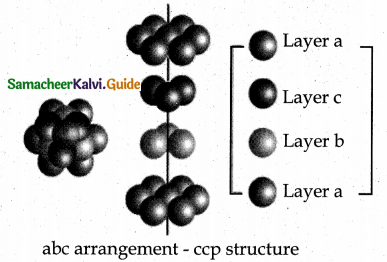Octahedral void:
A hole or void formed by three spheres of a hexagonal layer and another three spheres of the adjacent layer is a hole between six spheres. The spheres are arranged at the vertices of a regular octahedron. Such a hole or void is abc arrangement – ccp structure called octahedral void.Question 13.
Why ionic crystals are hard and brittle?
The ionic compounds are very hard and brittle. In ionic compounds, the ions are rigidly held in a lattice because the positive and negative ions are strongly attracted to each other and difficult to separate. But the brittleness of a compound is now easy to shift the position of atoms or ions in a lattice.

If we apply pressure on the ionic compounds the layers shifts slightly. The same charged ions in the lattice come closer. A repulsive force arises between same charged ions, due to this repulsion the lattice structure breaks down chemical bonding.

Question 14.
Calculate the percentage efficiency of packing in case of a body-centered cubic crystal. Packing efficiency.
In the body-centered cubic arrangement, the spheres are touching along the leading diagonal of the cube as shown in the In ∆ABC,
AC2 = AB2 + BC2
AC = $$g\sqrt { { AB }^{ 2 }+{ BC }^{ 2 } }$$
AC = $$g\sqrt { { a }^{ 2 }+{ a }^{ 2 } }$$ = a2In ∆ACG,
AG2 = AC2 + CG2
AG =$$g\sqrt { { AC }^{ 2 }+{ CG }^{ 2 } }$$
AG = $$\sqrt { { \left( \sqrt { 2 } a \right) }^{ 2 }+{ a }^{ 2 } }$$
AG = $$g\sqrt { { 2a }^{ 2 }+a^{ 2 } }$$ = $$g\sqrt { 3a^{ 2 } }$$ = $$\sqrt { 3 } a$$
i.e., $$g\sqrt { 3 }$$a = 4r
r = $$\frac { \sqrt { 3 } }{ 4 } a$$
∴ Volume of the sphere with radius ‘r’
= $$\frac { 4 }{ 3 }$$ πr2
= $$\frac { 4 }{ 3 }$$ π $$\left( \frac { \sqrt { 3 } }{ 4 } a \right)$$
= $$\frac { \sqrt { 3 } }{ 6 }$$ πa2
Number of spheres belong to a unit cell in bee arrangement is equal to two and hence the total volume of all spheres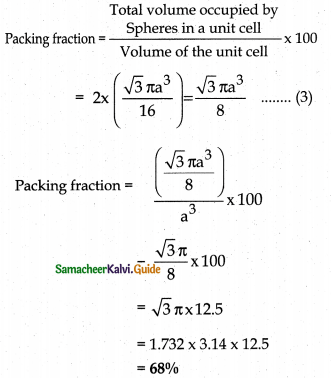i.e., 68% of the available volume is occupied.The available space is used more efficiently than in simple cubic packingQuestion 15.
What is the two-dimensional coordination number of a molecule in square close-packed layer?
Square close packing – When the spheres of the second row are placed exactly above those of the first row. This way the spheres are aligned horizontally as well as vertically. The arrangement is AAA type. Coordination number is 4.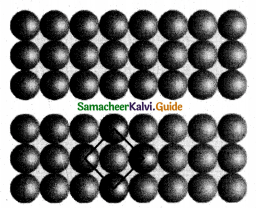Question 16.
What is meant by the term “coordination number”? What is the coordination number of atoms in a bcc structure?

• Coordination number – The number of nearest neighbours that surrounding a particle in a crystal is called the coordination number of that particle.
• Coordination number of atoms in a bcc structure is 8

Question 17.
An element has bcc structure with a cell edge of 288 pm. the density of the element is 7.2 gcm-3. How many atoms are present in 208g of the element.
An elemeñt has bec structure with a cell edge of 288 pm. The density of the element is 7.2 gcm-3. For the Bec structure, n = 2No. of atoms = No. of moles × Avagadro number
=n × NA
=4 × 6.023 × 1023
= 2.4092 × 1024 number of atomsQuestion 18.
Aluminium crystallizes in a cubic close-packed structure. Its metallic radius is 125pm. Calculate the edge length of the unit cell.
Cubic close packing is based on face centred cubic unit cell.
For fcc structure r = $$\frac{a \sqrt{2}}{4}$$
Given, Radius (r) = 125 pm
Edge length of unit cell (a) = ?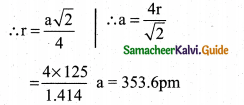Question 19.
If NaCI is doped with 10-2 mol percentage of strontium chloride, what is the concentration of cation vacancy?
We know that two Na+ ions are replaced by each of the Sr2+ ions while SrCl2, is doped with NaCI. But in this case, only one lattice point is occupied by each of the Sr2+ ions and produce one cation vacancy.

Here 10-2 mole of SrCl2, is doped with 100 moles of NaCI. Thus, cation vacancies produced by NaCi = 10-2  mol. Since, 100 moles of NaCl produces cation vacancies after doping = 10-2 mol. Therefore, I mole of NaCl will produce cation vacancies after doping

= $$\frac { { 10 }^{ -2 } }{ 100 }$$= 10-4mol
:. Total cationic vacancies,
= 10 × 6.023 × 1023
= 6.023 × 1019 vacancies

Question 20.
KF crystallizes in fcc structure like sodium chloride, calculate the distance between K and F in KF. (given : density of KF is 2.48 g cm-3)
For fcc structure n = 4
p = 2.48 gcm-3
For KFM= 39+19= 58g mol-1
NA = 6.O23 × 1023; a = ?a3= 0.1553 × 10-21 cm3
a= 0.5375 × 10-7 cm
a = 5375 × 10-8 cm
Distance between K+ and F =
1/2 × edge length = 1/2 × a
= 1/2 × 5375 × 10-8
= 2.6875 × 10-8 cm
= 2.6875 × 10-10 m
= 268.75 × 10-12 m
= 268.75 pm

Question 21.
An atom crystallizes ¡n fcc crystal lattice and has a density of 10 gcm-3 with unit cell edge length of 100pm. calculate the number of atoms present in 1 g of crystal.
ρ = 10cm-3
NA = 6.023 × 1023
a = 100 Pm = 100 × 1012m
= 100 × 1010cm = 1.00 × 108cm
For fcc n = 4 M = ? w = 1g
$$\rho=\frac{n M}{a^{3} N_{A}}$$
$$M=\frac{\rho a^{3} N_{A}}{n}$$
$$\frac{10 \times\left(1.00 \times 10^{-8}\right)^{3} \times 6.023 \times 10^{23}}{4}$$
Molar mass M = 1.51g mol-1
Numberof moles n = $$\frac{W}{M}=\frac{1}{1.51}$$ = 0.6623moles
Number of atoms present inn moles = n x NA
Number of atoms. present in 0.6623 moles
=0.6623 × 6.023 × 1023
= 3.989 × 1023 number of atomsQuestion 22.
Atoms X and Y form bcc crystalline structure. Atom X is present at the corners of the cube and Y is at the centre of the cube. What is the formula of the compound?
Y – body centre atom 1 × 1/1 = 1
∴ The formula is X1Y1 or XY.

Question 23.
Sodium metal crystallizes in bcc structure with the edge length of the unit cell 4.3 × 10-8 cm. Calculate the radius of sodium atom.
Edge length of the unit cell (a) = 4.3 × 10-8cm
Radius of sodium atom (r) = ?
For bcc structure, r = $$\frac { \sqrt { 3 } }{ 4 } a$$
= $$\frac { \sqrt { 3 } }{ 4 } a$$ (4.3 × 10-8cm)
$$\frac { 1.732\times 4.3\times { 10 }^{ -8 } }{ 4 }$$
= $$\frac { 1.732 }{ 4 } \times { 10 }^{ -8 }cm$$
= 1.86 × 10-8 cm

Question 24.
Write a note on Frenkel defect.
This arises due to the dislocation of ions from its crystal lattice.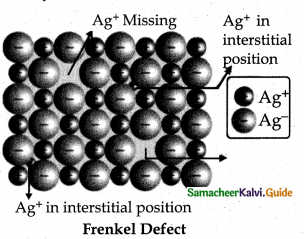• The ion missing from the lattice point occupies an interstitial position.
• This is shown by ionic solids in which cation and anion differ in size.
• This does not affect the density of the crystal eg. Ag Br
• Small Ag+ ion leaves its normal site and occupies an interstitial position.

III. Evaluate Yourself

Question 1.
An element has a face centered cubic unit cell with a length of 352.4 pm along an edge. The density of the element is 8.9 gcm-3. How many atoms are present in loo g of an element?
a = 352.4 pm 352. 4 × 10-12 m
= 352. 4 × 10-12 × 102 cm
= 352.4 × 10-10 cm
= 3.524 × 10-8cm
p = 8.9 gcm-3; NA 6.023 × 1023
Forfccn=4w= 100g ; M=?Molar mass M = 58.65 g mol-1
Number of moles
$$\mathrm{n}=\frac{\mathrm{w}}{\mathrm{m}}=\frac{100}{58.65}$$
= 1.705 moles
No of atoms present in n moles n. NA
No. of atoms present in 1.705 moles
= 1.705 × 6.023 × 1023
= 10.269 × 1024 number of atomsQuestion 2.
Determine the density of CsCl which crystallizes in a bcc type structure with an edge length 412.1 pm.
Molar mass of cscl = 168.5 g /mol
Number atoms present in per unit cell for bcc (cscl)
n = 1
Edge length (a) = 412.1 pm
Density (ρ) = ?
ρ = $$\frac { nM }{ { a }^{ 3 }{ N }_{ A } }$$Question 3.
A face centered cubic solid of an element (atomic mass 60) has a cube edge of 4 Å. Calculate its density.
For FCC unit cell n = 4
Edge length (a) = 4Å = 4 × 10-8cm
Mass (M) = 60 g mol-1
Density (p) = ?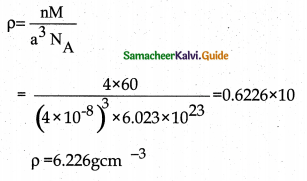Part – II – Additional Questions

Question 1.
In a crystalline solid the arrangement of its constituents is such that the potential energy of the system is
a) maximum
b) minimum
c) Zero
d) infinity
b) minimumQuestion 2.
The property of having identical values of physical properties in all directions is called as
a) isotropy
b) anisotropy
c) allotropy
d) isomerism
a) isotropy

Question 3.
The property of having different values of physical properties along different directions is called as
a) isotropy
b) anisotropy
c) allotropy
d) isomerism
b) anisotropyQuestion 4.
The radius of Na+ ion is 95pm and Cl” ion 181
pm. The co-ordination of Na+is f3Z9E3
a) 4
b) 6
c) 8
d) 3
b) 6

Question 5.
Amorphous solids show
a) isotropy
b) anisotropy
c) isomerism
d) tautomerism
a) isotropy

Question 6.
Amorphous solids are
a) anisotropic
b) true solids
c) pseudo solids
d) orderly arranged
c) pseudo solids

Question 7.
Crystalline solids are
a) isotropic
b) true solids
c) pseudo solids
d) super cooled liquids
b) true solidsQuestion 8.
In ionic solids, cations and anions are held together by
a) weak vander waals force
b) strong electrostatic force
c) hydrogen bond
d) covalent bond
b) strong electrostatic force

Question 9.
Ionic solids conduct electricity in
a) crystalline state
b) solid state
c) amorphous state
d) molten state
d) molten state

Question 10.
In covalent solids, the constituents are
a) ions
b) atoms
c) molecules
d) metals
b) atoms

Question 11.
Which among the following is a covalent solid?
a) Naphthalene
b) Urea
c) Diamond
d) Glucose
c) DiamondQuestion 12.
The crystal with a metal deficiency defect is
a) NaCl
b) KCl
c) ZnO
d) FeO
d) FeO

Question 13.
In molecular solids the constituents are held together by
a) weak vander waals force
b) strong electrostatic force
c) hydrogen bond
d) covalent bond
a) weak vander waals force

Question 14.
In non polar molecular solids the constituents are held together by
a) strong electrostatic force
b) Polar covalent bonds
c) London forces
d) hydrogen bonds
c) London forces

Question 15.
In polar molecular solids the constituents are held together by
a) strong electrostatic force
b) Polar covalent bonds
c) London forces
d) hydrogen bonds
b) Polar covalent bonds

Question 16.
Solid CO2 is a
a) Non polar molecular solid
b) Polar molecular solid
c) Hydrogen bonded molecular solid
d) Metallic solid
b) Polar molecular solidQuestion 17.
Naphthalene is a
a) Non polar molecular solid
b) Polar molecular solid
c) Hydrogen bonded molecular solid
d) Metallic solid
a) Non polar molecular solid

Question 18.
The number of close packed spheres is ‘n’. The number of tetrahedral voids generated is equal to ………
a) n
b) 2n
c) 2n2
d) 3n
b) 2n

Question 19.
In metallic solids, the lattice points are occupied by
a) anions
b) protons
c) atoms
d) metal ions
d) metal ioris

Question 20.
The melting point of metallic solids is
a) low
b)high
c) zero
d) negative
b) highQuestion 21.
For a rhombohedral crystal which of the following is correct?
a) α = β = γ = 90°
b) α = β = γ ≠ 90°
c) α = β = 90°; γ = 12090°
d) α = γ = 90°; β ≠ 90°
b) α = β = γ ≠ 90°

Question 22.
Each atom in the corner of a cubic unit cell is shared by …………….. neighbouring unit cells
a) 6
b) 8
c) 4
d) 2
b) 8

Question 23.
The body centred atom in a bcc unit cell is shared by …………….. unit cells
a) 1
b) 2
c) 4
d) 8
a) 1Question 24.
The face centred atom in a fee unit cell is shared by ………….. unit cells
a) 1
b) 2
c) 4
d) 8
b) 2

Question 25.
In FCC unit cell of the edge length is 8√2 pm. The radius of the metal atom is.
a) 0.04
b) 0.02
c) 8 × 10-2
d) 8/√2
a) 0.04

Question 26.
In fee, the face centre has a share of…….. of an atom1/2

Question 27.
Total number of atoms present in a simple cubic unit cell is
a) 1
b) 2
c) 4
d) 6
a) 1

Question 28.
Total number of atoms present in a bcc unit cell is
a) 1
b) 2
c) 4
d) 6
b) 2

Question 29.
Total number of atoms present in a fee unit cell is
a) 1
b) 2
c) 4
d) 6
c) 4Question 30.
of all the metals in the periodic table only …………… crystallizes in simple cubic pattern.
a) Uranium
b) Polonium
c) Titanium
b) Polonium

Question 31.
Which of the following is amorphous?
a) Poly Styrene
b) Table salt
c) Silica
d) Diamond
a) Poly Styrene

Question 32.
The arrangement of crystallographic axes and angles respectively in hexagonal crystal system is
a) a ≠ b ≠ c α = β = γ = 90°
b) a = b ≠  c α = β = γ = 90°
c) a = b ≠  c α = β = 90°; γ = 120°
d) a = b = c α ≠ β ≠ γ = 90°;
c) a = b ≠  c α = β = 90°; γ = 120°

Question 33.
For tetrahedral coordination number, the radius ratiois
a) 0.732 -1.000
b) 0.414 – 0.732
c) 0.225 – 0.414
d) 0.155 – 0.225
c) 0.225 – 0.414

Question 34.
If ‘a’ stands for the edge length of the cubic systems, SC, bcc, fee then the ratio of radii of the spheres in these systems will be respectively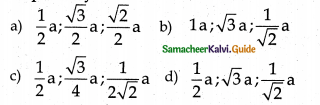cQuestion 35.
The arrangement ABC ABC ABC ……….. is referred as
a) Tetergonal close packing
b) hexagonal close packing
c) Octahderal close packing
d) Cubic close packing
d) Cubic close packing

Question 36.
Hexagonal close packed arrangement of ions is described as
a) ABC ABA
b) ABC ABC….
c) AB AB
d) ABB ABB ….
c) AB AB

Question 37.
An ionic compound has a unit cell consisting of A ions at the corners of a cube and B ions on the centres of the faces of the cube. The empirical formula of this compound would be
a) AB
b) A2B
c) AB3
d) A3B
c) AB3

Corner atom A $$8 \times \frac{1}{8}=1$$
Face centre atoms B $$6 \times \frac{1}{2}$$ = 3
∴ Formula is A1B3

Question 38.
In a fee lattice A type atoms are present at the corners while B type atoms are at face centres. If in each unit cell, one of the A type atom is missing from the corner what is the simplest formula of the compound
a) AB3
b) A3B
c) A7B24
d) A24B7
Ans:
c) A7B24
Corner atom A (one missing) = 7 x 1/8 = 7/8
Face centre atom B = 6 x 1/2 = 3
∴ Formula is A7/8 , B3 (or) A7 B24Question 39.
In a face centred cubic lattice, atoms A occupies the corner and B occupies the face centre. If one atom of B is missing from one of the face centred position, What is the formula of the compound?
a) A2B
b) AB2
c) A2B3
d) A2B5
d) A2B5
Corner atom A 8 × 1/8 = 1
Face centre atom B (one missing) 5 × 1/2 = 5/2
∴ Formulais A1B5/2 g, (or)A2B5

Question 40.
In Bragg’s equation, n represents
a) number of moles
b) Principal quantum number
d) Order of reflection
d) Order of reflection

II. Match the following

Question 1.

 Solid Example i. Covalent Solid a) NaCl ii. Molecular Solid b) Copper iii. Ionic Solid c) Graphite iv. Metallic Solid d) Dry ice

i. Graphite
ii. Dry ice
iii. NaCl
iv. CopperQuestion 2.

 Unit cells Number of atoms i. Simple cubic a) Four ii. body centred cubic b) One iii. face centered cubic c) Two

i. One
ii. Two
iii. Four

Question 3.

 Unit cell Packing fraction i. Simple cubic 68% ii. body centred cubic 74% iii, face centreI cubic 52.31%

i. 52.31%
ii. 68%
iii. 74%

Question 4.

 Point defect Example i. Schottky defect a. FeO ii. Frenkel defect b. NaCl in sodium vapour AgBr iii. Metal excess defect c. NaCl iv. Metal deficiency defect d. AgBr

1. NaCl
2. AgBr
3. NaCl in sodium vapour AgBr
4. FeO

III. Tick the odd man out

1. a) Plastics
b) Rubber
c) NaCl
d) Glass
c) NaCl
Reason : NaCl is a crystalline solids while others are amorphous solids.2. a) Naphthalene
b) Silicon carbide
c) Solid CO2
d) Urea
b) Silicon carbide
Reason : Silicon carbide is a covalent solid while others are molecular solids.

3. a) NaCl
b) AgBr
c) FeO
d) VO
c) FeO
Reason : FeO is a crystal with non stoichiometric defect while others are crystals with stoichiometric defect.

IV. Pick out the correct statements.

Question 1.
i) In covalent solids atoms are bound together by covalent bonds.
ii) Covalent solids are hard.
iii) Covalent solids have low melting point
iv) Covalent solids are good thermal and electrical conductors.
a) (i) & (ii)
b) (ii) & (iii)
c) (iii) & (iv)
d) (i) & (iv)
Correct statement:
a) (i) & (ii)
iii) Covalent solids have high melting point.
iv) Covalent solids are poor thermal and electrical conductors.Question 2.
i) A unit cell that contains only one lattice point is called a primitive unit cell.
ii) There are seven primitive crystal systems.
iii) Based on the seven primitive unit cell, Bravais defined 41 possible crystal systems.
iv) For cubic and rhombohedral crystal systems a b c.
a) (i) & (ii)
b) (ii) & (iii)
c) (iii) & (iv)
d) (i) & (iv)
Correct statement:
a) (i) & (ii)
iii) Based on the seven primitive unit cell, Bravais defined 14 possible crystal systems
iv) For cubic and rhombohedral crystal systems a = b = c.

Question 3.
i) In bcc unit cell the coordination number of each atom is 6.
ii) Density of a unit cell = $$\frac{\mathrm{a}^{3} \mathrm{~N}_{\mathrm{A}}}{\mathrm{nM}}$$
iii) Packing fraction =
$$\frac{\text { Total volume occupied by atoms } / \text { spheres }}{\text { Volume of the unit cell }} \times 100$$
iv) Stoichiometric defect in ionic solid is also
called as intrinsic or thermodynamic defect,
a) (i) & (ii)
b) (ii) & (iii)
c) (iii) & (iv)
d) (i) & (iv)
Correct statement:
c) (iii) & (iv)
i) In bcc unit cell the coordination number of each atom is 8.
ii) Density of a unit cell = $$\frac{\mathrm{nM}}{\mathrm{a}^{3} \mathrm{~N}_{\mathrm{A}}}$$

Question 4.
i) According to the law of nature, crystals must be perfect.
ii) Imperfection in solids affect the physical and chemical properties of the solid.
iii) Doping with impurity leads to a crystal imperfection.
iv) Imperfection decreases the electrical conductivity of a semi conductor
a) (i) & (ii) b) (ii) & (iii)
c) (iii) & (iv) d) (i) & (iv)
Correct statement:
b) (ii) & (iii)
i) According to the law of nature crystals need not be perfect.
iv) Imperfection increases the electrical conductivity of a semi conductor

V. Pick out the incorrect statement.

Question 1.
i) Solids have definite volume and shape.
ii) Solids are rigid and compressible
iii) Solids have weak cohesive forces.
iv) Solids have short inter atomic distances,
a) (i) & (ii)
b) (ii) & (iii)
c) (iii) & (iv)
d) (i) & (iv)
Correct statement:
b) (ii) & (iii)
ii) Solids are rigid and incompressible.
iii) Solids have strong cohesive forces.Question 2.
i) In molecular solids the constituents are neutral molecules.
ii) In molecular solids the constituents are held together by strong electrostatic force.
iii) Molecular solids are hard.
Molecular solids do not conduct electricity,
a) (i) & (ii)
b) (i),(ii) & (iii)
c) (ii) & (iv)
d) (ii) only
Correct statement:
d) (ii) only
(ii) In molecular solids the constituents are held together by weak Vanderwaals force.

Question 3.
i) Crystalline solids have short range, random
arrangement of constituents.
ii) Amorphous solids are isotropic.
iii) Crystalline solids are considered as pseudo solids.
iv) Heat of fusion of amorphous solids is not definite.
a) (i)&(ii)
b) (i) & (iii)
c) (iii) & (iv)
d) (i) & (iv)
Correct statement:
b) (i) & (iii)
i) Crystalline solids have long range orderly arrangement of constituents,
iii) Crystalline solids are considered as true solids.

Question 4.
i) The inter planar distance between two successive planes of atoms can be calculated using Bragg’s equation.
ii) Bragg’s equation is 2d sinθ = nλ.
iii) The coordination number of the sphere in simple cubic arrangement is 8.
iv) If the number of close packed spheres be ‘n’ then the number of octahedral voids is equal to ‘2n’.
a) (i) & (ii)
b) (i) & (iii)
c) (iii) & (iv)
d) (i) & (iv)
Correct statement:
c) (iii) & (iv)
(iii) The coordination number of the sphere in simple cubic arrangement is 6.
(iv) If the number of close packed spheres be ‘n’ then the number of octahedral voids is equal to’n’.

VI. Assertion and Reason

Question 1.
Assertion A : Ionic solids do not conduct electricity in solid state.
Reason : Ionic solids have high melting points.
i) Both A and R are correct and R explains A.
ii) A is correct but R is wrong. iii) A is wrong but R is correct,
iv) Both A and R are correct and R does not explain A.
iv) Both A and R are correct and R does not explain A. Correct Reason: In ionic solids ions are fixed in the Lattice positions.Question 2.
Assertion A : Graphite is a component of many lubricants.
Reason R : Graphite is slippery.
i) Both A and R are correct and R explains A.
ii) A is correct but R is wrong. iii) A is wrong but R is correct,
iv) Both A and R are correct and R does not explain A.
i) Both A and R are correct and R explains A.

Question 3.
Assertion A : Metal deficiency defect arises due to the presence of less number of anions than the cations.
Reason R : This defect is observed in a crystal in which, the cations have variable oxidation states.
i) Both A and R are correct and R explains A.
ii) A is correct but R is wrong. iii) A is wrong but R is correct,
iv) Both A and R are correct and R does not explain A.
iii) A is wrong but R is corrrect
Correct Assertion(A): Metal deficiency defect arises due to the presence of less number of cations than the anions.

Question 4.
Assertion A : In polar molecular solids, the constituents are molecules formed by polar covalent bonds.
Reason R : They are held together by relatively weak dipole – dipole interactions.
i) Both A and R are correct and R explains A.
ii) A is correct but R is wrong.
iii) A is wrong but R is correct.
iv) Both A and R are correct and R does not explain A.
ii) A is correct but R is wrong.
Correct Reason R : They are hold together by relatively strong dipole – dipole interactions.

VII. Two Mark Questions.

Question 1.
What is a crystalline solid?
A crystalline solid is one in which its constituents (atoms,ions or molecules) have an orderly arrangement over a long range.

Question 2.
What is an amorphous solid?
An amorphous solid is one in which its constituents (atoms, ions or molecules) have random arrangement over a short range.Question 3.
Define isotropy.

• Isotropy means uniformity in all directions.
• Isotropy means having identical values of physical properties in all directions. (ex): Amorphous solids show isotropy.

Question 4.
What is meant by anisotropy?

• Anisotropy means non uniformity in all directions.
• Anisotropy means having different values of physical properties in different directions.
(ex): Crystalline solids show anisotropy.

Question 5.
What are molecular solids?

• Solids in which the structural units are neutral molecules are known as molecular solids.
• The neutral molecules are held together by weak VanderWaals force.
• They are generally soft.
• They do not conduct electricity.

Question 6.
What are non – polar molecular solids?

• Molecular solids in which neutral molecules are held together by weak dispersion forces or London forces are known as non – polar molecular solids.
• They have low melting point.
• They are usually liquids or gases at room temperature.
• (ex): naphthalene, anthracene.

Question 7.
What are polar molecular solids?

• Solids in which the constituents are molecules formed by polar covalent bonds are called polar molecular solids.
• The molecules are held together by relatively strong dipole – dipole interactions.
• They have higher melting point than non
polar molecular solids, (ex): Solid CO2, Solid NH3.

Question 8.
Outline the classification of the point defects.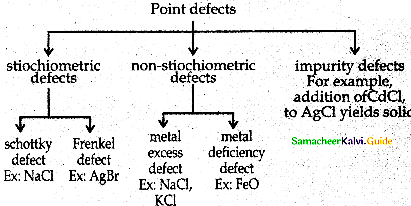• To maximize the attractive force, cations are surrounded by as many anions as possible and vice versa.
• They posses definite crystal structure.
• Many ionic solids are cubic close packed, (ex): NaCl crystal.Question 4.
What are covalent solids?

• Solids in which the structural units are atoms are known as covalent solids.
• The atoms are bound together by covalent bonds in a three dimensional network.
• They are very hard.
• They have high melting point.
• They are usually poor thermal and electrical conductors, (ex): Diamond, Silicon Carbide.

Question 5.
Write about simple cubic unit cell.

• In the simple cubic unit cell each corner is occupied by identical atoms, ions or molecules.
• They touch along the edges of the cube.
• They do not touch diagonally.
• The coordination number is 6.
• Each atom in the corner is shared by 8 neighbouring unit cells.
• Number of atoms in a SC unit cellQuestion 6.
Write about body centred cubic (bcc) unit cell.

• In bcc unit cell each corner is occupied by identical atoms, ions or molecules.
• In addition one atom occupies the body centre.
• The atoms in the corners do not touch each other.
• However they all touch the atom present in the body centre.
• Each atom is surrounded by eight nearest neighbours. and coordination number is 8.
• The atom at the body centre belongs to only one unit cell =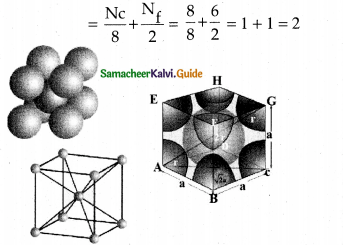Question 7.
Sketch face centred cubic (fee) unit cell and calculate the number of atoms present in it.

• In fcc unit cell each corner is occupied by identical atoms, ions or molecules.
• In addition one atom occupies the centre of eachface.
• The atoms in the corners do not touch each other.
• However they all touch the atoms present at the centre of the faces.
• The coordination number is 12.
• Number of atoms in a fee unit cell =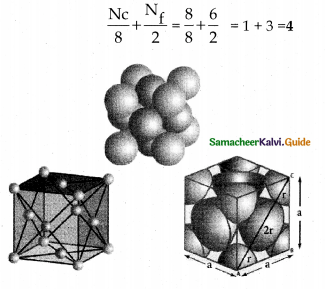Question 8.
What is radius ratio in ionic solid? Tabulate the relation between radius ratio and structural arrangement in ionic solids.
The ratio of radius of cation and anion plays an important role in determining the structure.
Radius ratio = $$\frac{r_{C}+}{r_{A-}}$$IX. Additional Questions – 5 Mark

Question 1.
What is Bragg’s equation. Using Bragg’s equation how can you calculate the edge of the unit cell.
X – ray diffraction analysis is the most powerful tool for the determination of crystal structure.
The interplanar distance (d) between two successive planes of atoms can be calculated using the following Bragg’s equation from ray diffraction data.
2d sinθ = nλ
λ = wave length of x – ray used for diffraction
θ = angle of di fTraction
n = order of reflection (diffraction)
d = interplanar distance
By knowing the values of θ, λ and n we can calculate the value of d.
$$\mathrm{d}=\frac{\mathrm{n} \lambda}{2 \sin \theta}$$
Using those values the edge of the unit cell can be calculated.Question 2.
How can you calculate the density of the unit cell of a crystal?
Using the edge length of a unit cell we can calculate the density of the crystal by considering a cubic unit cell as follows.
Density of unit cell p = $$\frac{\text { mass of the unit cell }}{\text { Volume of the unit cell }}$$ ……………1
Mass of the unit cell = Total number of atoms present in the unit cell × Mass of one atom ………….2
Mass of an atom=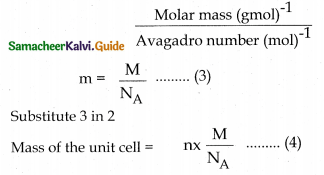For a cubic unit cell all the edge lengths are equal ie ; a = b = c
Volume of the unit cell = a × a × a = a3. …..5
Substituting 4 and 5 in 1
Density of the unit cell p = $$\frac{\mathrm{nM}}{\mathrm{a}^{3} \mathrm{~N}_{\mathrm{A}}}$$ …..6
From the values of n, M, a and NA, the density of the unit cell of the crystal can be calculated.

Question 3.
Calculate the percentage efficiency of packing in case of a simple cubic crystal.
Let us consider a cube with an edge length ‘a’.
Volume of the cube = a × a × a = a3.
Let ‘r’ is the radius of the sphere.
From the figure a = 2r; r = a/2
Volume the sphere (atom)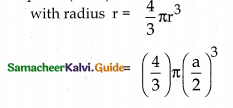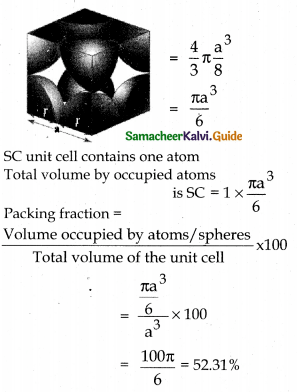ie ; only 52.31% of the available volume is occupied by atoms (spheres) in SC crystal.Question 4.
Calculate the percentage efficiency of packing in case of face centred cubic crystal.
Let us consider a fee crystal.
In ∆ABC AC2 = AB2 + BC2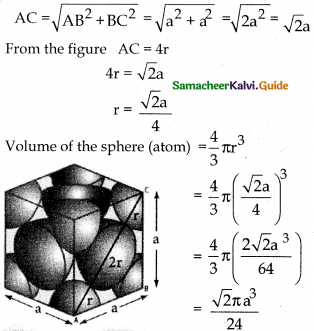ie; only 74% of the available volume is occupied by atoms (spheres) in fee crystal.

Question 1.
X – ray diffraction studies show that an element crystallises in an fee unit cell with edge length 360.8pm. If density of the element is 8.92gcm-3 , calculate its atomic mass.
a 360.8pm = 360.8 × 10-12 m
= 360.8 × 10-10cm =3.608 × 10-8Cm
p = 8.92 g cm-3 fee unit cell n = 4
NA = 6.023 × 1023 M=?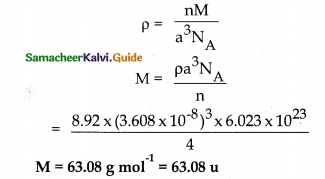Question 2.
Silver forms ccp lattice and x – ray studies of its crystals show that edge length of its unit cell is 408.6 pm. If the atomic mass of silver is 107.9 u calculate its density.
Solution:
Cubic close packing is based on face centred cubic unit cell n = 4
a = 408.6pm = 408.6 × 10-12
m = 408.6 × 10-10 cm
= 4.086 × 10-8 cm
M = 107.9u = 107.9 gmol-1 NA=6.023 × 1023
p = ?p = 10.50 g cm-3

Question 3.
An element with molar mass 27 g mol-1 forms a cubic unit cell with edge length 405pm. If its density is 2.7 g cm-3, what is the nature of the cubic unit cell?
M = 27 g mol-1
a = 405pm = 405 × 10-12 m = 405 × 10-10cm
= 4.05 × 10-8cm
p = 2.7 gem-3NA = 6.023 × 1023 n =?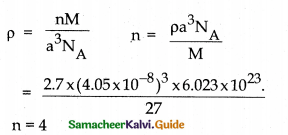Since one unit cell contains 4 atoms it is face centred cubic unit cell.

Question 4.
Niobium crystallises in body centred cubic structure, if its density is 8.55g cm’3 and atomic mass is 93 u calculate its atomic radius,
For bcc n = 2 p = 8.55 g cm-3
M = 93 gmol-1 NA = 6.023 × 1023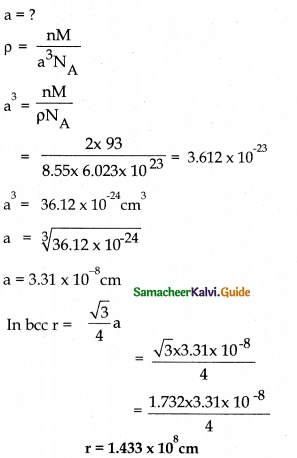Question 5.
Gold with atomic radius 0.144 nm crystallises in face centred cubic unit cell. What is the length of a side of the cell?
r = 0.144 a = ?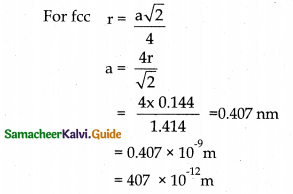Edge length a = 407 pm.Question 6.
Aluminium crystallises in cubic close packed structure. Its metallic radius is 125pm. (i) Calculate the edge length of the unit cell, ii) How many unit cells are there in 1cm3 of aluminium?
r = 125pm a = ?
Cubic dose packed structure is based on face centred cubic unit cell.
For fee n =4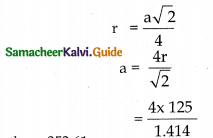Edge length a = 353.61pm

ii) 22400cm3 contain 6.023 × 1023 number of atoms
1 cm3 contains = $$\frac{6.023 \times 10^{23}}{22400} \times 1$$
= 2.689 × 1019 number of atoms,
In fcc
4 atoms occupy 1 unit cell
2.689 × 1019 atoms occupy 1/4 × 2.689 × 1019 unit cells
=6.723 × 1018 unit cells
1 cm3 of aluminium contains 6.723 × 1018 unit cells

Question 7.
The diffraction of crystal of Barium with x – ray of wave length 2.29Å gives a first order reflection at 27°81 . What is the distance between the diffracted planes?
n = 1 λ= 2.29Å
θ = 27°81
d = ?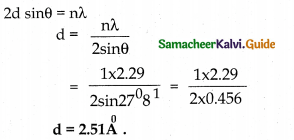Question 8.
A certain solid crystallises in SC lattices first order x – ray ( λ = 0.154nm) reflection maximum from a set of (200) planes was observed at 16°61. Calculate the edge of the unit cell.